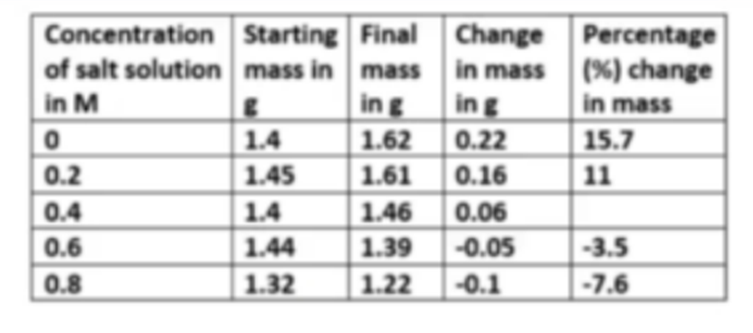Calculate the % change in mass for 0.4m salt solution

What is the independant variable for this investigation?

What is the dependent variable for this investiagtion?

Why is the percentage change in mass the variable used for the graph?

Why should you test at each concentration at least three times?

Which of these would improve the accuracy of the results for this investigation?